# Convert Column Number to Letter – Excel & Google Sheets

This tutorial will demonstrate how to convert a column number to its corresponding letter in Excel.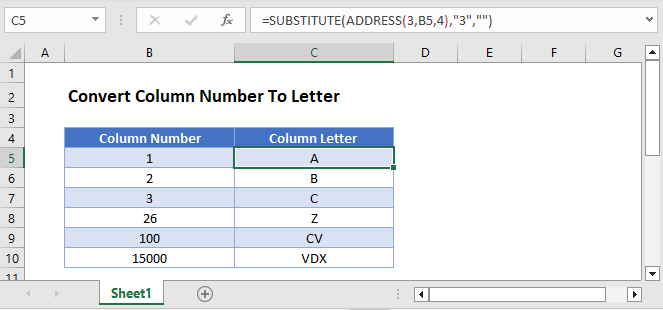To convert a column number to letter we will use the ADDRESS and the SUBSTITUTE Functions.

``=SUBSTITUTE(ADDRESS(3,B3,4),"3","")``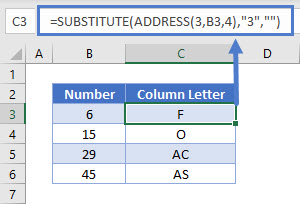The formula above returns the column letter in the position of the number in the referenced cell.

Let’s walk through the above formula.

First, we will use the ADDRESS Function to return a cell address based on a given row and column.

``=ADDRESS(3,B3,4)``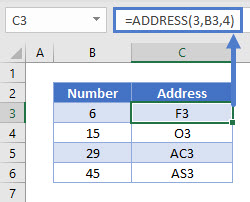Important! You must set the [abs_num] input to 4, otherwise the cell address will display with \$-signs.

## The SUBSTITUTE Function

Next, we use the SUBSTITUTE Function to replace the row number “3” with a blank string of text (“”) leaving only the column letter.

``=SUBSTITUTE(C3,"3","")``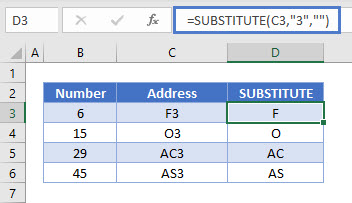Putting the functions together results to the initial formula, which converts the column numbers into letters.

Please note that any number can be used as the row number within the ADDRESS Function as long as you enter the same row number into the SUBSTITUTE Function.

## Convert Column Number to Letter in Google Sheets

These formulas work exactly the same in Google Sheets as in Excel.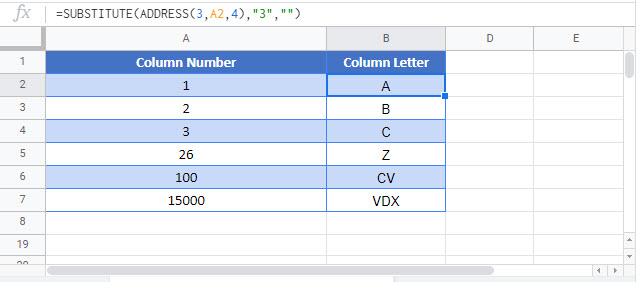### Excel Practice Worksheet

Practice Excel functions and formulas with our 100% free practice worksheets!